The transformer is the main point of power that can step down to use the device like as lightnings, motors, Air-conditioning, printer, computer…etc.

Here is the calculation of the transformer’s power that we known already about:

AMPS: Amperage or Amps provided by an electrical service is the flow rate of electrical current that is accessible. Accurately, Amps = Watts / Volts. (Amps = Watts divided by Volts)

Volts: Volt, properly, is defined as the potential difference across a conductor when a current of one ampere dissipates one watt of power that conductor is able to delivering more energy to the user. Accurately Volts = Watts / Amps. (Volts equals Watts divided by Amps).

KVA: KVA stands for Kilo Volt Amps, which is calculated by a volt and amp meter. KVA is for clear power. kVA is used to compute what size wiring you need, what size breaker, etc, to power a circuit of a given amperage/wattage. KVA rating is higher than the wattage on an AC circuit.

Transformer’s Power Calculation for 3 Phase Voltage

In case, We know I :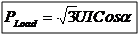that: U = 400V, I = 100A, Cos? = 0.8

So, PLoad = 55,425.60 W, Then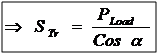Then we get,  STr = 69,282.00 VA

The other way, we calculate I easy way that we know STr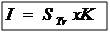That K = 1.44, Why we say K equal 1.44, because of K = 1000/(400*1.73)

and  STr = 69,282.00 VA = 69.282 kVA

so we get I = 99.8A

Transformer’s Power Calculation for 1 Phase Voltage

In case, we know I :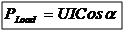that U = 230V, I = 100A, Cos? = 0.8

So, PLoad = 18,400.00 W, Then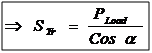Then we get,  STr = 23,000.00 VA

The other way, we calculate I easy way that we know STr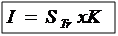That K = 4.34, Why we say K equal 4.34, because of K = 1000/230

and  STr = 23,000.00 VA = 23 kVA

So we get I = 99.8 A